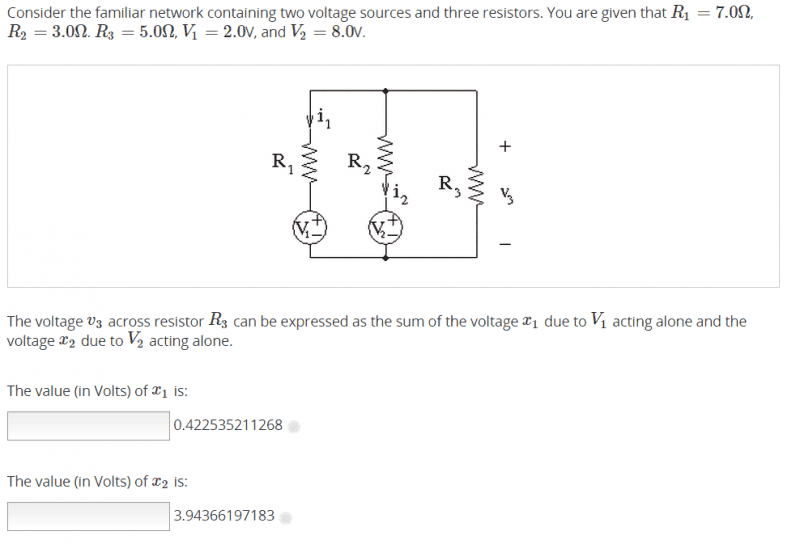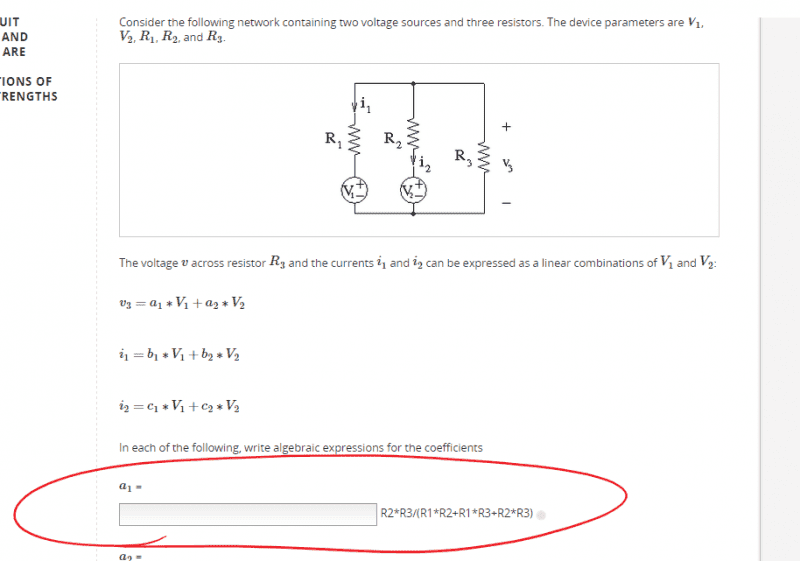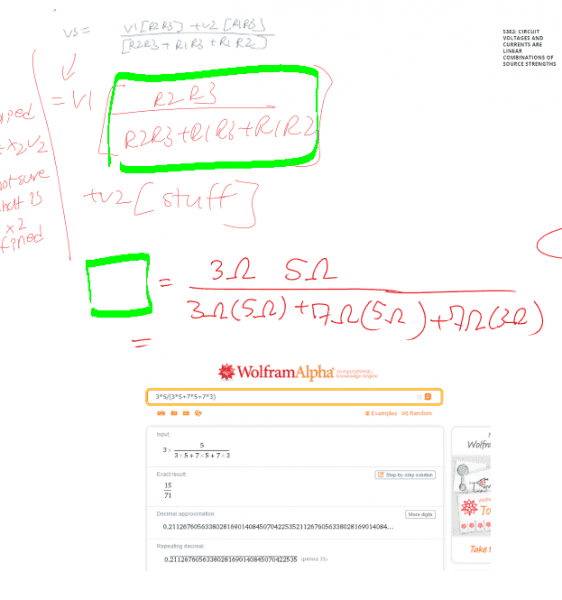# What does "sum of voltage x1 due to v1" mean?

• LongApple
In this particular problem, x1 is the voltage due to V1 acting alone and x2 is the voltage due to V2 acting alone.f

## Homework StatementV=IR

## The Attempt at a Solution

My first guess is the quote in the title of this thread meant the a1 except now a1 * v3 instead of a1 in the below image, which we already have from a previous problem. I think my interpretation may be wrongI think that my interpretation may be wrong. I try to solvee for a1 and get 0.21. So I thought x1= a1 * v3 = 0.21 * v3

but v3 is not about 2 Volts so I have made a mistake#### Attachments

I thought x1= a1 * v3
No. "V1 acting alone" means x1 is the value v3 would have if v2 were 0.

## Homework Statement

View attachment 77960

V=IR

## The Attempt at a Solution

My first guess is the quote in the title of this thread meant the a1 except now a1 * v3 instead of a1 in the below image, which we already have from a previous problem. I think my interpretation may be wrong

View attachment 77958

I think that my interpretation may be wrong. I try to solvee for a1 and get 0.21. So I thought x1= a1 * v3 = 0.21 * v3

but v3 is not about 2 Volts so I have made a mistake

View attachment 77959

As you apply the typical loop rule sum, you can treat V3 as a sum of I1 * R3 and I2 * R3 ,
where the rest of the loop voltages come from V2 - I2 * R2 , or else V1 - I1 * R1 .
that treats the node almost like a switch, choosing the left branch or the right branch.

Here, they want one Voltage source, or the other ... that is,
if you short out V2 (=0), so that V1 supplies R1 in series with R3 || R2 ;
this would make the voltage across the parallel pair = V1 (R2||R3)/(R1+(R2||R3))
... so I agree that , a1=0.211
if you short out V1 , then V2 supplies R2 in series with R3 || R1,
this would make V3 = 0.493 V2 ... so in the end, V3 = 4.366 Volt

The phrase you should be querying is "the voltage x1 due to V1 acting alone."

It means the voltage of interest is the sum of a number of independent components. One component we shall call x1 and another component shall be called x2. These can be calculated separately, then added to find the actual voltage of interest.